# Javascript Function: Arrow function

by Ridwan Fadilah, updated on Apr 22, 2020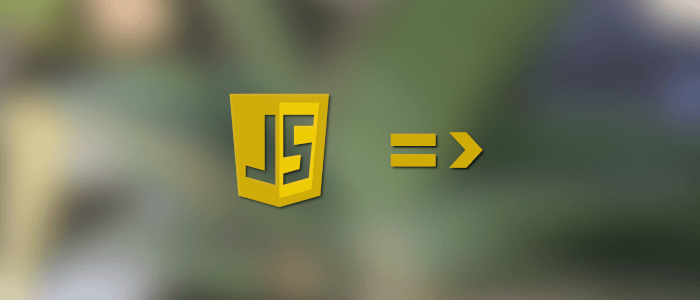#### Tutorial to knowing about the usage of Javascript arrow function, example, and definition

In the previous tutorials, we've seen examples of javascript functions. Now, in this tutorial, we'll learn about another function usage in javascript known as an arrow function. In my opinion, this arrow function is so cool, especially if we're talking about the efficiency of code writing in a program.

## Definition

An arrow function expression is a syntactically compact alternative to a regular function expression. - MDN

### How to make an Arrow Function In Javascript?

Before that, let's see the function expression usage first:

``````    // Function Expression

const myFunction = function (name) {
return `Hello, \${name}`;
}

console.log(myFunction('Ridwan')); ``````

To make these function expression to be an arrow function, see the basic syntaxis below:

``    const functionName = (param) => { return `String literal, \${param}`; }``

Note: The arrow function is using a template/string literal. Template literals are enclosed by the backtick (` `).

Example:

``````    <!DOCTYPE html>
<html lang="en">

<title>Document</title>

<body>

<script>
const myFunction = (name) => {
return `Hello, \${name}`;
}
console.log(myFunction('Ridwan'));
</script>
</body>

</html>``````

Result: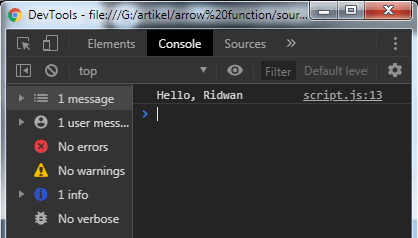If you have more than one parameter, you can use these following syntax:

``````    <!DOCTYPE html>
<html lang="en">

<title>Document</title>

<body>

<script>
const myFunction = (time, name) => {
return `Good \${time}, \${name}`;
}
console.log(myFunction('Mornig', 'Ridwan'));
</script>
</body>

</html>``````

Result: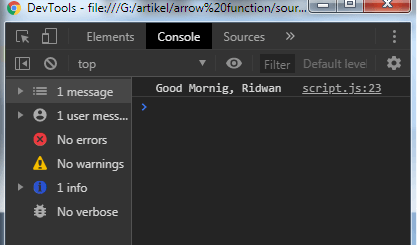If you only have one parameter and the function is only returning a value, you can make it more compact like this example:

``````    <!DOCTYPE html>
<html lang="en">

<title>Document</title>

<body>

<script>
// implicit return

const myFunction = name => `Hello, \${name}`;
console.log(myFunction('Ridwan'));
</script>
</body>

</html>``````

These called implicit return, and the arrow function still runs well the same as before: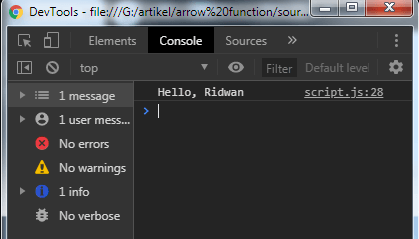And the last examples of the usage this arrow function is when the program doesn't have a parameter the arrow function must contain empty parentheses "( )" symbol.

Example:

``````    <!DOCTYPE html>
<html lang="en">

<title>Document</title>

<body>

<script>
const myFunction = () => `Hello World!`;
console.log(myFunction());
</body>

</html>``````

Result: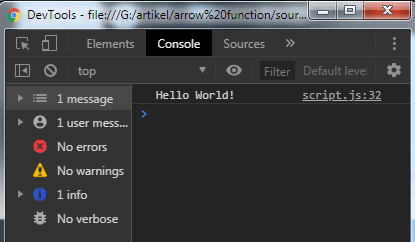## Arrow Function with Function Map

You've seen the basics of arrow functions usage before. Now, let's try another example of an arrow function with a function map in javascript. For example, we'll try to count all the characters on some names, and we'll try to change the result from returning an array and then make it as an object.

Example:

``````    <!DOCTYPE html>
<html lang="en">

<title>Document</title>

<body>

<script>
let peoples = ['Ridwan Fadilah', 'Jhon Due', 'Mike Wazowski'];
let allChar = peoples.map(name => name.length);
console.log(allChar);
</body>

</html>``````

And the result will display by the browser console like this: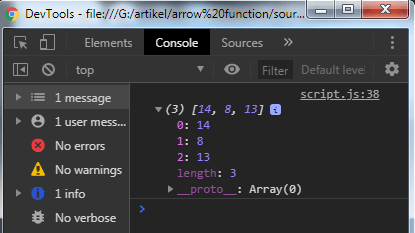To make it an object, you must use the curly braces and the parentheses "({ })"
I'll try to get the object name and counting all the characters of the names. In this case, the function also must have a property and fills by the parameter/argument.

``    (param => ({ property: param )})``

Here's the example:

``````    <!DOCTYPE html>
<html lang="en">

<title>Document</title>

<body>

<script>
let peoples = ['Ridwan Fadilah', 'Jhon Due', 'Mike Wazowski'];
let allChar = peoples.map(name => ({
name: name,
charLength: name.length
}));
console.log(allChar);
</body>

</html>``````

Result: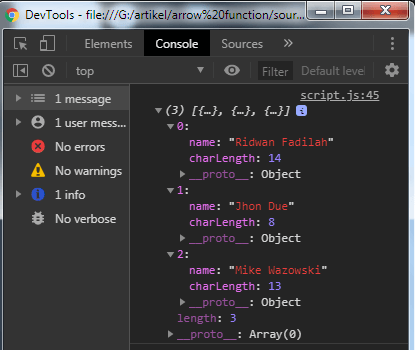In the newest javascript, you can remove the property name if the property same as the parameter.

Example:

``````    <!DOCTYPE html>
<html lang="en">

<title>Document</title>

<body>

<script>
let peoples = ['Ridwan Fadilah', 'Jhon Due', 'Mike Wazowski'];
let allChar = peoples.map(name => ({
name,
charLength: name.length
}));
console.table(allChar);
</body>

</html>``````

I use the "console.table()" to make it look good:## The "this" in Arrow Function

Arrow function does not have the 'this' concept. When we use the 'this' concept in a constructor function, it will work so well. In other functions, the 'this' will represent the object that could be the window, document, button or whatever.

It so different if we are using the arrow function. With arrow functions, the 'this' always represents the object that defined the arrow function.

Example:

``````    <!DOCTYPE html>
<html lang="en">

<title>Document</title>

<body>

<script>
const person = {
name: 'Ridwan',
sayHello: function () {
console.log(`Hello, my name is \${this.name}.`);
}
}
</body>

</html>``````

When we type and call the function 'person.sayHello()' there is no problem: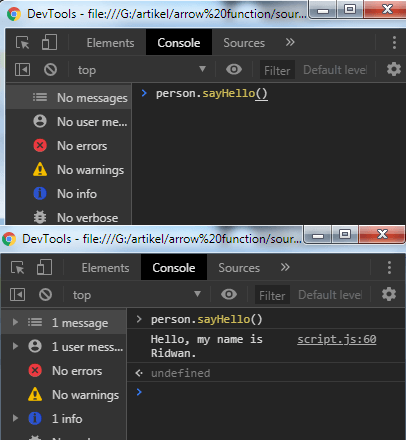And if the arrow function is used:

``````    <!DOCTYPE html>
<html lang="en">

<title>Document</title>

<body>

<script>
const person = {
name: 'Ridwan',
sayHello: () => {
console.log(`Hello, my name is \${this.name}.`);
}
}
</body>

</html>``````

The name is undefined or blank: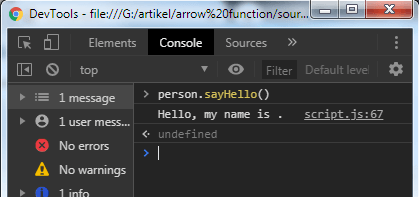That's just a simple way of arrow function usage. In the real case, we'll not be able to use it on a program with more high complexity.

That just the basic. If you need more deep learning about HTML, CSS, Javascript or related you can take the following cheap course:

• HTML & HTML5 For Beginners (A Practical Guide)• Web - HTML• Learn HTML, CSS, JAVASCRIPT• JavaScript:• Learn JavaScript Fundamentals• Learning JavaScript Shell ScriptingThanks!